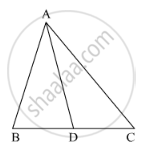Share

In the Following Figure, Check Whether Ad is the Bisector of ∠A of ∆Abc in the Following Case: Ab = 8 Cm, Ac = 24 Cm, Bd = 6 Cm and Bc = 24 Cm - CBSE Class 10 - Mathematics

Question

In the following figure, check whether AD is the bisector of ∠A of ∆ABC in the following case:

AB = 8 cm, AC = 24 cm, BD = 6 cm and BC = 24 cmSolution

It is given that, AB = 8 cm, AC = 24 cm, BD = 6 cm and BC = 24 cm

We have to check whether AD is bisector of ∠A.

First we will check proportional ratio between sides.

Now

DC = BC - BD

DC = 24 - 6 = 18

So "AB"/"AC"="BD"/"DC"

rArr8/24=6/18

rArr1/3=1/3

(It is proportional)

Hence, AD is bisector of ∠A.

Is there an error in this question or solution?

Video TutorialsVIEW ALL 

Solution In the Following Figure, Check Whether Ad is the Bisector of ∠A of ∆Abc in the Following Case: Ab = 8 Cm, Ac = 24 Cm, Bd = 6 Cm and Bc = 24 Cm Concept: Angle Bisector.
S